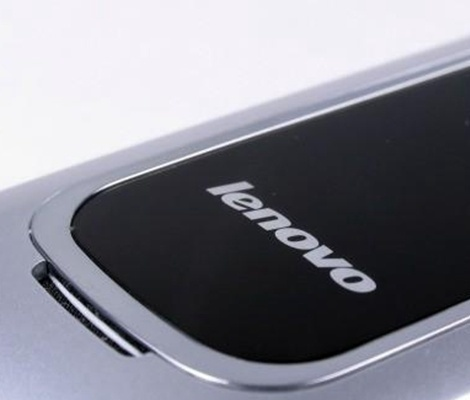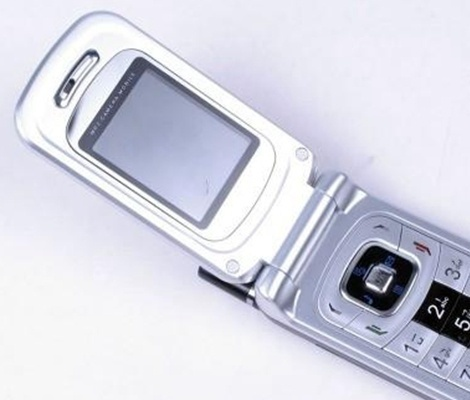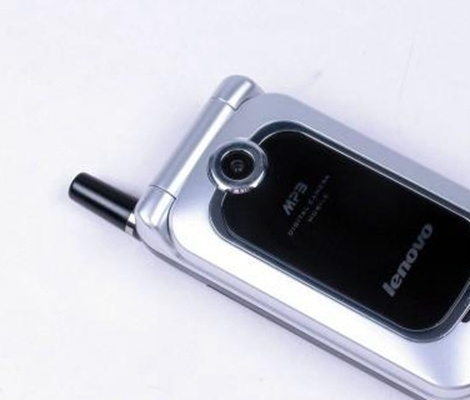|

# 联想e355价格与评测

2006年仍旧是诺基亚领跑的手机年代，在当时诺基亚作为每一个人心目中的机神的时候，其他品牌的手机想要成功地吸引消费者的目光是非常困难的，但是联想手机却做到的。联想e355是一款由联想公司在2006年上市发行的一款按键翻盖手机，这款手机采用周围白，中间黑的设计，黑白强对比的色彩设计，让人在第一眼看到它的时候就深深地被它吸引住了，再加上它那流畅的线条，对消费者是真的有强大的杀伤力。

联想e355价格

这款手机在2007年就停产了，停产前价格在600元左右。（价格来源网络，仅供参考）

联想e355评测

科技、时尚、简单、轻松和品质，成为联想手机愈发明显的产品特质。而通过充分的市场调研、发掘不同层次消费者的真实需求，强调用户体验将是联想手机2006财年实现超越目标的一柄利剑。在联想发布的9款春季手机新品中，E355作为一款时尚、简洁且功能实用的入门机型，凭借MP3+拍照的功能和超低的的价格率先登陆市场，成为今年4月联想在低端市场的主推产品。联想E355采用了时尚大气的折叠单屏设计，流线型的外观配黑色的中心色显得比较柔和，也符合手机目前的外观设计思路。机身正面曜石黑辉映着月光银，最经典的两种颜色结合起来显得简洁时尚、典雅大方。面壳材质明亮动人，手感也很不错，但是比较容易沾上指印等污迹。翻开手机看到的是符合人体工学的波浪型键盘设计，使操作更方便。按键手感非常好，用起来很舒服，几乎从来不会错误按键。独有的屏幕透明拨号功能，可显示每行7个字的大拨号字体，使设计更具人性化。特别适合视力不好的人尤其是老年人使用。网络上，手机支持GSM/GPRS，900/1800MHz双频，尺寸大小为85.3×43.4×20.3mm，重量也只有85克，不大也不小小手感刚刚好。通话质量很好，声音还原很真，没有杂音，打电话时声音大小可通过上下键调。屏幕方面，手机采用了1.6英寸的65536色TFT彩色屏幕，分辨率为128×160像素，显示效果相当不错。竞争对手同档次的手机都是CSTN屏幕，E355已经遥遥领先了。可设置墙纸，预置5种(2张动画，3张静态)，也可自定义，使用自拍墙纸;支持主菜单动画;待机主画面可设置运营商、时间和个性词语，可以像在MSN上每天起名一样了。

手机提供了500条名片式记录，可存储姓名，手机，家庭电话，公司电话，铃声等联系人信息。手机电话本分组管理，组名可自定义。支持手机和SIM卡电话簿记录互相复制、移动。可存储最近30个已拨/未接/已接/已拒来电记录。具有来电防火墙功能，自动拒接黑名单内的来电。手机可存500个SMS短信息，MMS彩信的存储空间为300KB，可最多10人群发;并支持20秒语音彩信。它的短信编写模式是我用过的各种手机中最方便实用的。而且还支持超长短信，可以一次编写最多350个汉字的长短信，可最多30人群发，对于平时喜欢短信聊天的人来说再适合不过了。联想E355内置30万像素高清晰摄像头，还独具匠心地支持最多15秒的无声视频拍摄，使连续摄影不再是高端机型的专利。而且拍摄的细节还是非常丰富的，支持变换场景模式和7级数字变焦，并且添加了乐趣横生的像框模式和连拍自拍功能，可以给用户带来足够的拍摄乐趣。2种照片尺寸可选(640*480，128*160);多种拍摄模式:拍照模式，录像模式，像框模式，延时自拍，多张连拍(仅支持128×160分辨率下的连拍);多种拍摄场景选择，室内、室外、夜间;7级数字变焦，支持特殊效果(标准/褐色/黑白/底片)。30万像素不算高，但实际使用中已经足足有余，其拍照效果和E320接近，甚至可以更好，晚上的拍照效果都还不错。

联想E355的标配含两块锂电池(680mAh)和一个旅行充电器，有的商家会同时赠送有数据线和耳机，请消费者购买之前向商家咨询清楚，以免后悔。电池的待机时间很长，晚上关机的话，一般使用频率下使用一个星期问题不大，真的是又方便又实用。这款手机虽然是在2006年出品的手机，但是拿到现在2016年来销售，还是有一定的市场的，因为这款手机先不管性能如何，就它那白外黑里的设计风格来看，就是非常吸引人眼球的优点，而且这款手机在2006年的时候就配备了一块20万像素的摄像头，这个配置在当时算的上是非常厉害的了，同时这款手机最难得的就是这款手机的价格还在1000元以下，这样的价格让很多的消费者都能够拥有这样的一部手机。

`声明：本文由入驻焦点开放平台的作者撰写，除焦点官方账号外，观点仅代表作者本人，不代表焦点立场错误信息举报电话： 400-099-0099，邮箱：jubao@vip.sohu.com，或点此进行意见反馈，或点此进行举报投诉。`A B C D E F G H J K L M N P Q R S T W X Y Z
A - B - C - D - E
• A
• 鞍山
• 安庆
• 安阳
• 安顺
• 安康
• 澳门
• B
• 北京
• 保定
• 包头
• 巴彦淖尔
• 本溪
• 蚌埠
• 亳州
• 滨州
• 北海
• 百色
• 巴中
• 毕节
• 保山
• 宝鸡
• 白银
• 巴州
• C
• 承德
• 沧州
• 长治
• 赤峰
• 朝阳
• 长春
• 常州
• 滁州
• 池州
• 长沙
• 常德
• 郴州
• 潮州
• 崇左
• 重庆
• 成都
• 楚雄
• 昌都
• 慈溪
• 常熟
• D
• 大同
• 大连
• 丹东
• 大庆
• 东营
• 德州
• 东莞
• 德阳
• 达州
• 大理
• 德宏
• 定西
• 儋州
• 东平
• E
• 鄂尔多斯
• 鄂州
• 恩施
F - G - H - I - J
• F
• 抚顺
• 阜新
• 阜阳
• 福州
• 抚州
• 佛山
• 防城港
• G
• 赣州
• 广州
• 桂林
• 贵港
• 广元
• 广安
• 贵阳
• 固原
• H
• 邯郸
• 衡水
• 呼和浩特
• 呼伦贝尔
• 葫芦岛
• 哈尔滨
• 黑河
• 淮安
• 杭州
• 湖州
• 合肥
• 淮南
• 淮北
• 黄山
• 菏泽
• 鹤壁
• 黄石
• 黄冈
• 衡阳
• 怀化
• 惠州
• 河源
• 贺州
• 河池
• 海口
• 红河
• 汉中
• 海东
• I
• J
• 晋中
• 锦州
• 吉林
• 鸡西
• 佳木斯
• 嘉兴
• 金华
• 景德镇
• 九江
• 吉安
• 济南
• 济宁
• 焦作
• 荆门
• 荆州
• 江门
• 揭阳
• 金昌
• 酒泉
• 嘉峪关
K - L - M - N - P
• K
• 开封
• 昆明
• 昆山
• L
• 廊坊
• 临汾
• 辽阳
• 连云港
• 丽水
• 六安
• 龙岩
• 莱芜
• 临沂
• 聊城
• 洛阳
• 漯河
• 娄底
• 柳州
• 来宾
• 泸州
• 乐山
• 六盘水
• 丽江
• 临沧
• 拉萨
• 林芝
• 兰州
• 陇南
• M
• 牡丹江
• 马鞍山
• 茂名
• 梅州
• 绵阳
• 眉山
• N
• 南京
• 南通
• 宁波
• 南平
• 宁德
• 南昌
• 南阳
• 南宁
• 内江
• 南充
• P
• 盘锦
• 莆田
• 平顶山
• 濮阳
• 攀枝花
• 普洱
• 平凉
Q - R - S - T - W
• Q
• 秦皇岛
• 齐齐哈尔
• 衢州
• 泉州
• 青岛
• 清远
• 钦州
• 黔南
• 曲靖
• 庆阳
• R
• 日照
• 日喀则
• S
• 石家庄
• 沈阳
• 双鸭山
• 绥化
• 上海
• 苏州
• 宿迁
• 绍兴
• 宿州
• 三明
• 上饶
• 三门峡
• 商丘
• 十堰
• 随州
• 邵阳
• 韶关
• 深圳
• 汕头
• 汕尾
• 三亚
• 三沙
• 遂宁
• 山南
• 商洛
• 石嘴山
• T
• 天津
• 唐山
• 太原
• 通辽
• 铁岭
• 泰州
• 台州
• 铜陵
• 泰安
• 铜仁
• 铜川
• 天水
• 天门
• W
• 乌海
• 乌兰察布
• 无锡
• 温州
• 芜湖
• 潍坊
• 威海
• 武汉
• 梧州
• 渭南
• 武威
• 吴忠
• 乌鲁木齐
X - Y - Z
• X
• 邢台
• 徐州
• 宣城
• 厦门
• 新乡
• 许昌
• 信阳
• 襄阳
• 孝感
• 咸宁
• 湘潭
• 湘西
• 西双版纳
• 西安
• 咸阳
• 西宁
• 仙桃
• 西昌
• Y
• 运城
• 营口
• 盐城
• 扬州
• 鹰潭
• 宜春
• 烟台
• 宜昌
• 岳阳
• 益阳
• 永州
• 阳江
• 云浮
• 玉林
• 宜宾
• 雅安
• 玉溪
• 延安
• 榆林
• 银川
• Z
• 张家口
• 镇江
• 舟山
• 漳州
• 淄博
• 枣庄
• 郑州
• 周口
• 驻马店
• 株洲
• 张家界
• 珠海
• 湛江
• 肇庆
• 中山
• 自贡
• 资阳
• 遵义
• 昭通
• 张掖
• 中卫

1室1厅1厨1卫1阳台

1
2
3
4
5

0
1
2

1

1

0
1
2
3报名成功，资料已提交审核A B C D E F G H J K L M N P Q R S T W X Y Z
A - B - C - D - E
• A
• 鞍山
• 安庆
• 安阳
• 安顺
• 安康
• 澳门
• B
• 北京
• 保定
• 包头
• 巴彦淖尔
• 本溪
• 蚌埠
• 亳州
• 滨州
• 北海
• 百色
• 巴中
• 毕节
• 保山
• 宝鸡
• 白银
• 巴州
• C
• 承德
• 沧州
• 长治
• 赤峰
• 朝阳
• 长春
• 常州
• 滁州
• 池州
• 长沙
• 常德
• 郴州
• 潮州
• 崇左
• 重庆
• 成都
• 楚雄
• 昌都
• 慈溪
• 常熟
• D
• 大同
• 大连
• 丹东
• 大庆
• 东营
• 德州
• 东莞
• 德阳
• 达州
• 大理
• 德宏
• 定西
• 儋州
• 东平
• E
• 鄂尔多斯
• 鄂州
• 恩施
F - G - H - I - J
• F
• 抚顺
• 阜新
• 阜阳
• 福州
• 抚州
• 佛山
• 防城港
• G
• 赣州
• 广州
• 桂林
• 贵港
• 广元
• 广安
• 贵阳
• 固原
• H
• 邯郸
• 衡水
• 呼和浩特
• 呼伦贝尔
• 葫芦岛
• 哈尔滨
• 黑河
• 淮安
• 杭州
• 湖州
• 合肥
• 淮南
• 淮北
• 黄山
• 菏泽
• 鹤壁
• 黄石
• 黄冈
• 衡阳
• 怀化
• 惠州
• 河源
• 贺州
• 河池
• 海口
• 红河
• 汉中
• 海东
• I
• J
• 晋中
• 锦州
• 吉林
• 鸡西
• 佳木斯
• 嘉兴
• 金华
• 景德镇
• 九江
• 吉安
• 济南
• 济宁
• 焦作
• 荆门
• 荆州
• 江门
• 揭阳
• 金昌
• 酒泉
• 嘉峪关
K - L - M - N - P
• K
• 开封
• 昆明
• 昆山
• L
• 廊坊
• 临汾
• 辽阳
• 连云港
• 丽水
• 六安
• 龙岩
• 莱芜
• 临沂
• 聊城
• 洛阳
• 漯河
• 娄底
• 柳州
• 来宾
• 泸州
• 乐山
• 六盘水
• 丽江
• 临沧
• 拉萨
• 林芝
• 兰州
• 陇南
• M
• 牡丹江
• 马鞍山
• 茂名
• 梅州
• 绵阳
• 眉山
• N
• 南京
• 南通
• 宁波
• 南平
• 宁德
• 南昌
• 南阳
• 南宁
• 内江
• 南充
• P
• 盘锦
• 莆田
• 平顶山
• 濮阳
• 攀枝花
• 普洱
• 平凉
Q - R - S - T - W
• Q
• 秦皇岛
• 齐齐哈尔
• 衢州
• 泉州
• 青岛
• 清远
• 钦州
• 黔南
• 曲靖
• 庆阳
• R
• 日照
• 日喀则
• S
• 石家庄
• 沈阳
• 双鸭山
• 绥化
• 上海
• 苏州
• 宿迁
• 绍兴
• 宿州
• 三明
• 上饶
• 三门峡
• 商丘
• 十堰
• 随州
• 邵阳
• 韶关
• 深圳
• 汕头
• 汕尾
• 三亚
• 三沙
• 遂宁
• 山南
• 商洛
• 石嘴山
• T
• 天津
• 唐山
• 太原
• 通辽
• 铁岭
• 泰州
• 台州
• 铜陵
• 泰安
• 铜仁
• 铜川
• 天水
• 天门
• W
• 乌海
• 乌兰察布
• 无锡
• 温州
• 芜湖
• 潍坊
• 威海
• 武汉
• 梧州
• 渭南
• 武威
• 吴忠
• 乌鲁木齐
X - Y - Z
• X
• 邢台
• 徐州
• 宣城
• 厦门
• 新乡
• 许昌
• 信阳
• 襄阳
• 孝感
• 咸宁
• 湘潭
• 湘西
• 西双版纳
• 西安
• 咸阳
• 西宁
• 仙桃
• 西昌
• Y
• 运城
• 营口
• 盐城
• 扬州
• 鹰潭
• 宜春
• 烟台
• 宜昌
• 岳阳
• 益阳
• 永州
• 阳江
• 云浮
• 玉林
• 宜宾
• 雅安
• 玉溪
• 延安
• 榆林
• 银川
• Z
• 张家口
• 镇江
• 舟山
• 漳州
• 淄博
• 枣庄
• 郑州
• 周口
• 驻马店
• 株洲
• 张家界
• 珠海
• 湛江
• 肇庆
• 中山
• 自贡
• 资阳
• 遵义
• 昭通
• 张掖
• 中卫• 手机• 分享
• 设计
免费设计
• 计算器
装修计算器
• 入驻
合作入驻
• 联系
联系我们
• 置顶
返回顶部